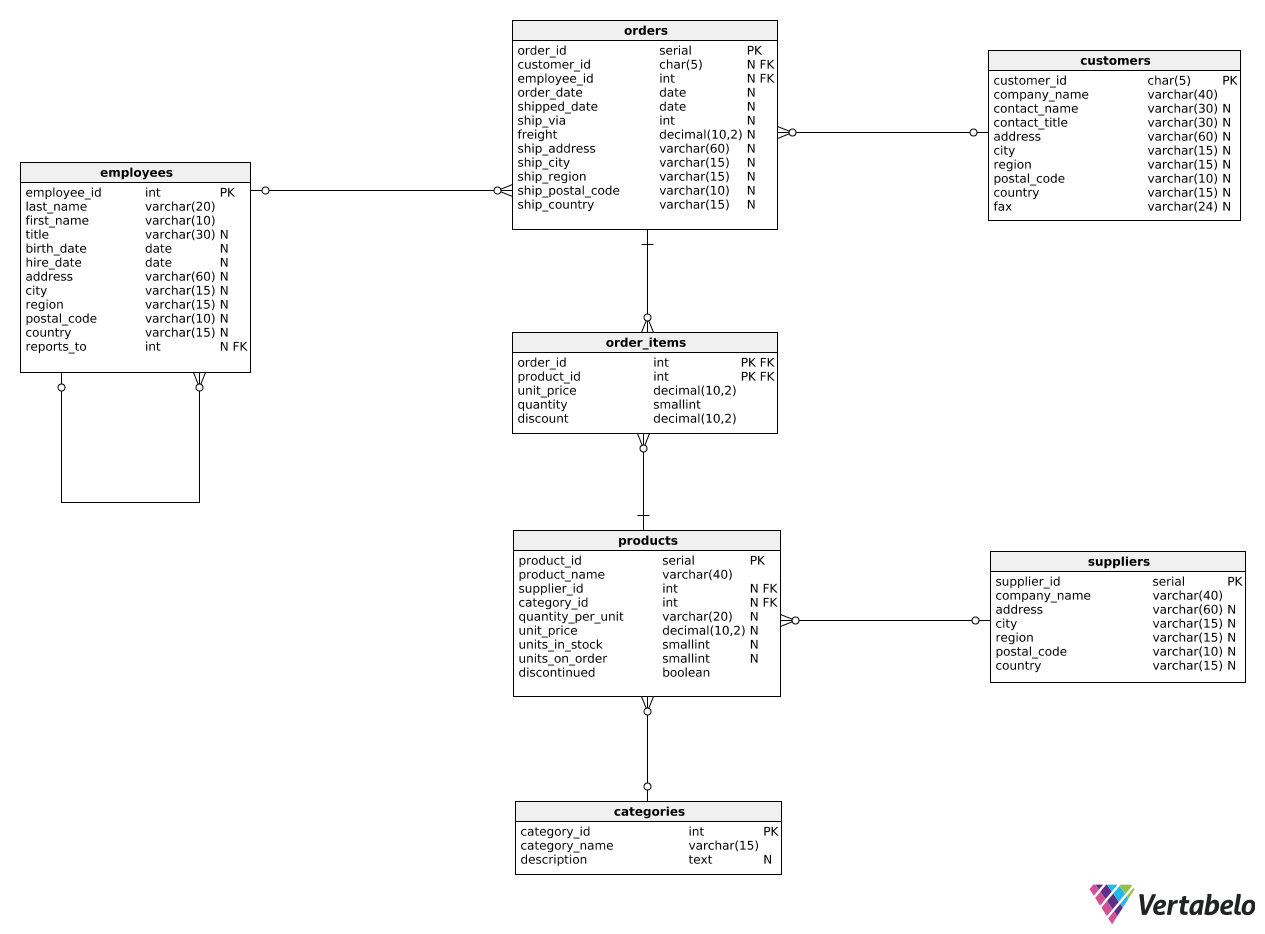Deals Of The Week - hours only!Up to 80% off on all courses and bundles.-Close
Introduction
Multiple metrics for a single object
2. Multiple metrics for a single object – explanation
Metrics for two groups
Ratios and percentages
Global vs. specific metrics
Summary

## Instruction

We will start with a simple report that shows multiple metrics for the same business object. Let's say we need to show the number of products and the total price for each order. Take a look at the query below:

SELECT
order_id,
COUNT(product_id) AS products,
SUM(unit_price * quantity) AS total_price
FROM order_items
GROUP BY order_id;


We use COUNT() and SUM() in two separate columns to show two different metrics for the same business object (i.e., for the same order). In this case, we used the order_items table to show the number of line items and the total price for each order. Note that we use the GROUP BY statement to compute metrics for different orders in one query.

## Exercise

We want to see each customer's ID alongside the number of orders they placed and the total revenue (after discount) that their purchases generated generated for us. Show three columns:

• The customer's ID (customer_id).
• The number of orders (as order_count).
• The total price paid for all orders after discounts (as total_revenue_after_discount).

### Stuck? Here's a hint!

Join three tables: orders, order_items, and customers. Remember to group by customer_id and to use DISTINCT when counting the number of orders. Calculate the total_revenue_after_discount column using SUM(unit_price * quantity * (1 - discount)).